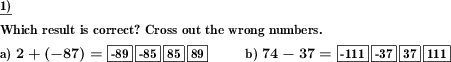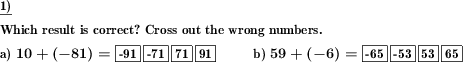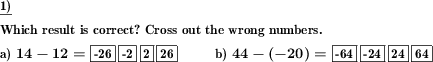Custom math worksheets at your fingertips# Details for problem "Negative whole numbers add and subtract choose result"

Quickname: 8902

Elementary School, Primary School, Junior High School, Middle School, High School.

## Summary

For an addition or subtraction problem with negative numbers, the correct result must be chosen.

## Examples## Description

Arithmetic with negative numbers. Problems are presented either as addition or subtraction tasks or mixed.

The number of problems and the number space can be chosen. To simplify the problems slightly, you can specify that the first summand will always be positive.

Four possible results are then presented for selection. The correct number has to be determined, the other numbers have to be crossed out.

Download free printable worksheets for this math problem here. The worksheet contains the problems only, the solution sheet includes the answers. Just click on the respective link.

•Worksheet 1Solution sheet with answers
•Worksheet 2Solution sheet with answers
•Worksheet 3Solution sheet with answers

If you can not see the solution sheets for download, they may be filtered out by an ad blocker that you may have installed. If this is the case, please allow ads for this page and reload the page. The solution sheets will then reappear.

• Do these sample worksheets do not really fit?
• Do you need more math worksheets, with a different level of difficulty?
• Would you like to combine different problems on a worksheet and adjust them to your needs?
• As a teacher, you can put together your own worksheets using the automatically generated math problems provided.
With a free initial credit, you can start creating your own math worksheets in a few minutes.

You can try it for free! Register here, to create custom worksheets now!

## Customization options for this problem

Parameter
Possible values
Number of problems
1, 2, 3, 4, 5, 6, 7, 8, 9, 10
Number range
20, 30, 40, 50, 100, 200, 500, 1000, 10000, 100000
Mixed, Plus, Minus
First summand negative
never, can be

## Similar problems

Remark
Description
Also exists without choice of possible results
Negative whole numbers are to be added and subtractedDeutsche Version dieser Aufgabe
These informational pages with samples describe math problems that can be combined on custom math worksheets with solutions for home and K-12 school use.
Deutsche Seiten
×